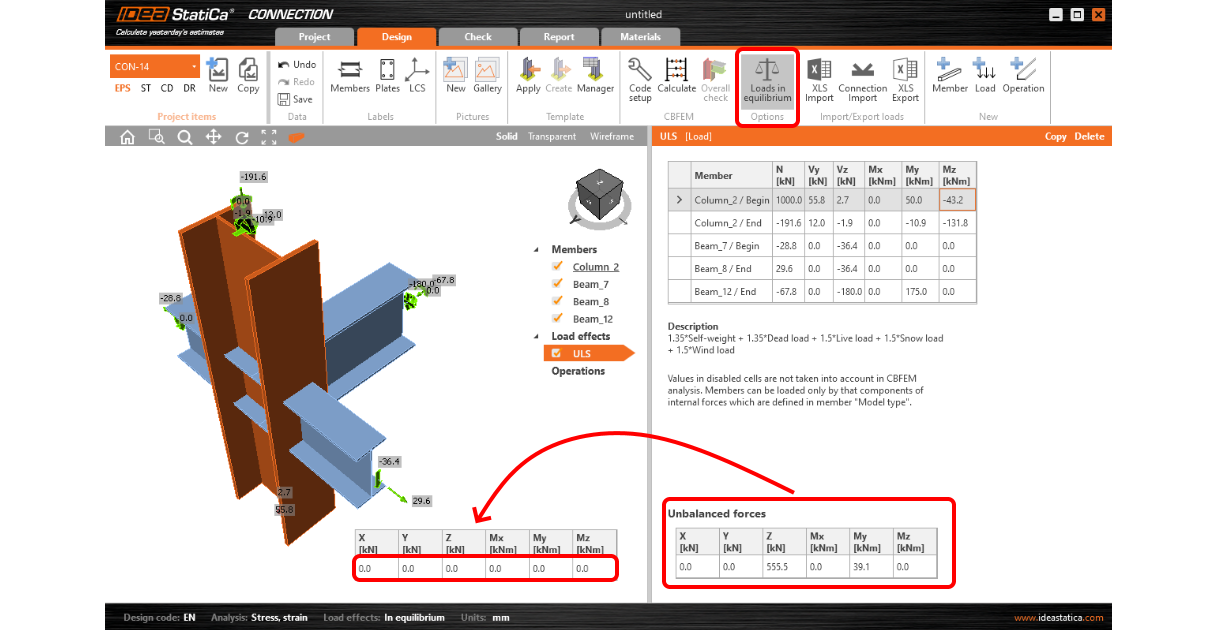### Alegeți limba# Unbalanced forces from BIM link import

SupportCenter.LocalizedArticle
$$When you use a BIM link to load data from FEA programs to IDEA StatiCa, the algorithm finds the values of selected load cases/combinations/classes in the node and performs the import of forces. Given the law of mechanics, the equilibrium of the internal forces in the node must be present. Unfortunately, the equilibrium is sometimes not achieved after the import.Unbalance of internal forces after the import to IDEA StatiCa can happen in the following cases:Case: A point force is placed in the node imported to IDEA StatiCa. The software cannot detect which member should transfer this point force and, therefore, it is not taken into account in the analysis model.Solution: Do not use point forces in global analysis. If necessary, the force must be manually added to a selected member as a normal or shear force.Case: Loaded, non-steel (usually timber or concrete) member is connected to the imported node. Such a member is not considered in the analysis and its internal forces are ignored in the analysis.Solution: Replace the concrete member with a concrete block and anchorage.Case: The node is a part of a slab or a wall (usually from concrete). The slab or the wall is not a part of the model and its internal forces are ignored.Solution: Replace the concrete slab or wall with a concrete block and anchorage.Case: Some members are connected to the imported node via rigid links. Such members are not included in the model and their internal forces are ignored.Solution: Add these members into the list of connected members manually.Case: There is a seismic load in the structural model. The imbalance is caused by the nature of the calculation method widely used. In seismic states, the values of individual internal forces are calculated using the square root of the sum of powers and therefore they are all positive, and this way they are also imported.Solution: The user has to consider whether the effect of the imbalance can be accepted.$$